# Grade FG Positiontoterm rules Generate terms of a

• Slides: 10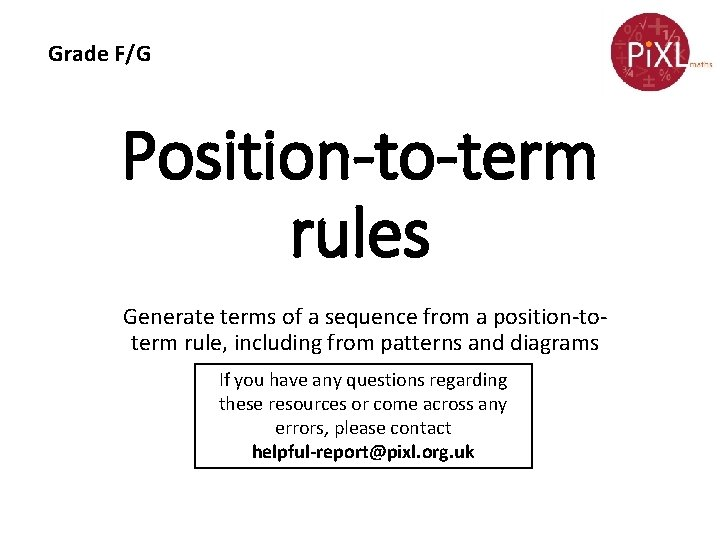Grade F/G Position-to-term rules Generate terms of a sequence from a position-toterm rule, including from patterns and diagrams If you have any questions regarding these resources or come across any errors, please contact [email protected] org. uk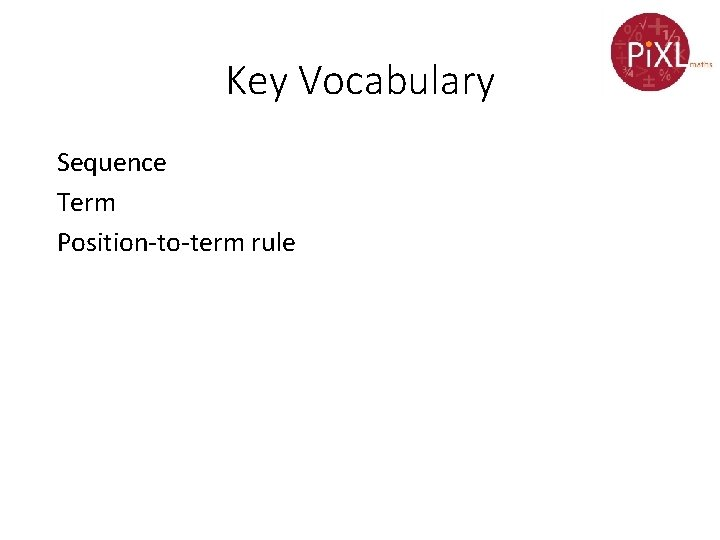Key Vocabulary Sequence Term Position-to-term rulePosition-to-term Rule The position-to-term rule are the operations applied to the position number of a term in a sequence in order to generate that term. Examples: Write down the first five terms of the sequence for each of these position-to-term rules. (a) Position number add 8 Position No 1 2 3 4 5 Add 8 9 10 11 12 13 (b) (3 x position number) subtract 2 Position No 1 2 3 4 5 Multiply 3 3 6 9 12 15 Subtract 2 1 4 7 10 13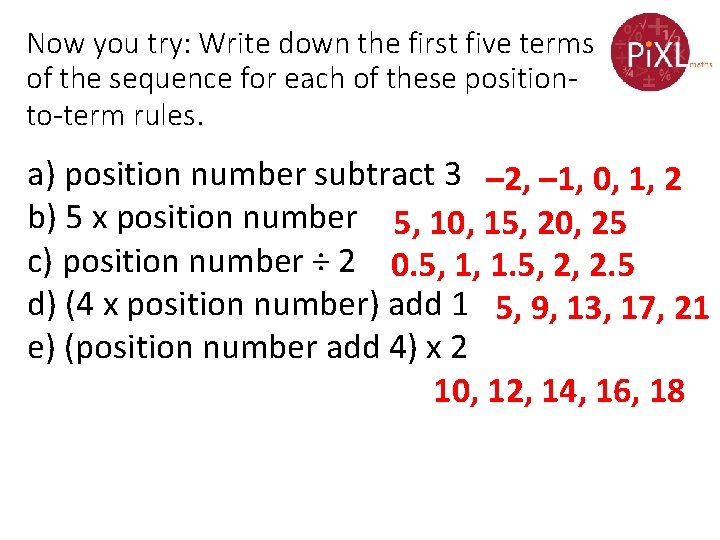Now you try: Write down the first five terms of the sequence for each of these positionto-term rules. a) position number subtract 3 – 2, – 1, 0, 1, 2 b) 5 x position number 5, 10, 15, 20, 25 c) position number ÷ 2 0. 5, 1, 1. 5, 2, 2. 5 d) (4 x position number) add 1 5, 9, 13, 17, 21 e) (position number add 4) x 2 10, 12, 14, 16, 18Position-to-term Rule The table shows the first five terms of a number sequence and their position in the sequence. (a) Describe the position-to-term rule Position No 1 2 3 4 5 Term 9 18 27 36 45 (b) Write down the 20 th term in the sequence Solution (a) The position-to-term rule is the position number multiplied by 9 or position number x 9 (b) The 20 th term is 20 x 9 =180Now you try: Write down the position-toterm rule for each of these sequences Position No 1 2 3 4 5 Term 12 13 14 15 16 position number add 11 Position No 1 2 3 4 5 Term 6 12 18 24 30 position number x 6 Position No 1 2 3 4 5 Term 3 5 7 9 11 (2 x position number) add 1 Position No 1 2 3 4 5 Term 9 19 29 39 49 (10 x position number) subtract 1Position-to-term Rule From Patterns Look at these shapes made from squares Shape 1 Shape 2 Shape 3 Shape 4 (a) Draw the 4 th shape. (b) Complete the table Shape No 1 2 3 4 5 No of squares 5 7 9 11 13 (c) Write down the position-to-term rule No. of squares = 2 x position number + 3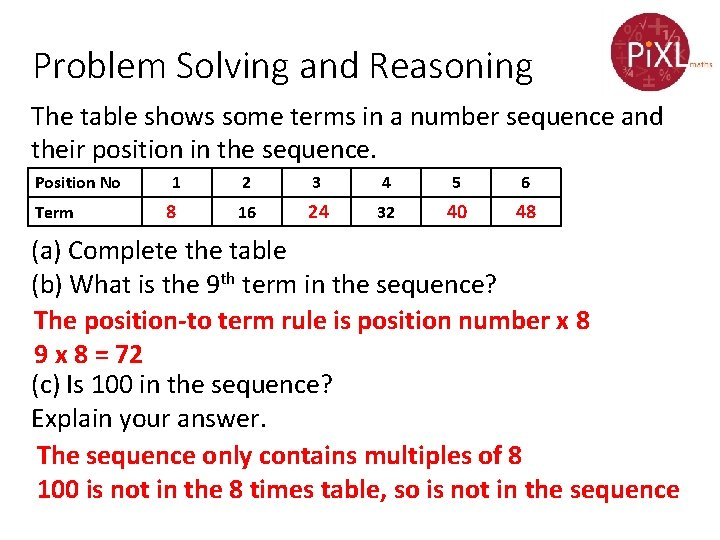Problem Solving and Reasoning The table shows some terms in a number sequence and their position in the sequence. Position No Term 1 8 2 3 4 5 6 16 24 32 40 48 (a) Complete the table (b) What is the 9 th term in the sequence? The position-to term rule is position number x 8 9 x 8 = 72 (c) Is 100 in the sequence? Explain your answer. The sequence only contains multiples of 8 100 is not in the 8 times table, so is not in the sequence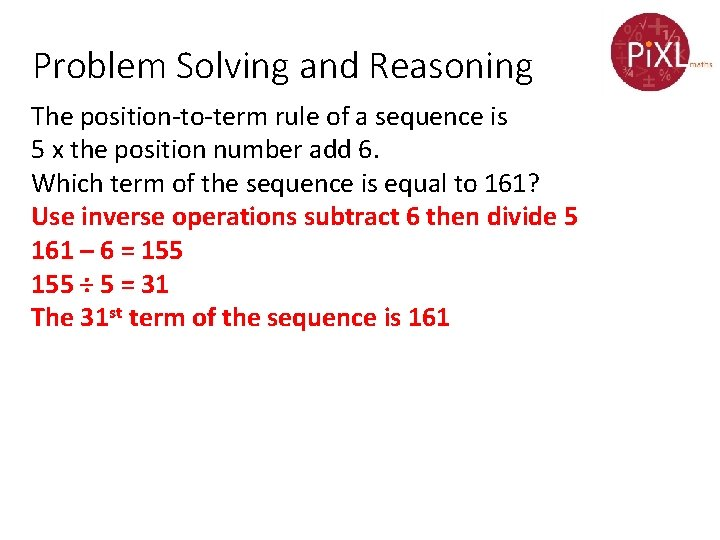Problem Solving and Reasoning The position-to-term rule of a sequence is 5 x the position number add 6. Which term of the sequence is equal to 161? Use inverse operations subtract 6 then divide 5 161 – 6 = 155 ÷ 5 = 31 The 31 st term of the sequence is 161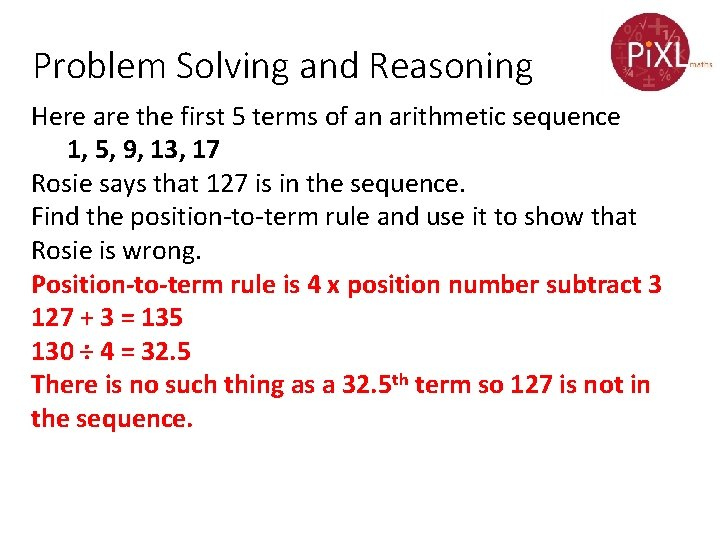Problem Solving and Reasoning Here are the first 5 terms of an arithmetic sequence 1, 5, 9, 13, 17 Rosie says that 127 is in the sequence. Find the position-to-term rule and use it to show that Rosie is wrong. Position-to-term rule is 4 x position number subtract 3 127 + 3 = 135 130 ÷ 4 = 32. 5 There is no such thing as a 32. 5 th term so 127 is not in the sequence.